# The moons dataset and decision surface graphics in a Jupyter environment – II – contourplots

I proceed with my present article series on the “moons dataset” as an example for classification tasks in the field of “machine learning” [ML]. My objective is to gather basic knowledge on Python related tools for performing related experiments. In my last blog article

The moons dataset and decision surface graphics in an Jupyter environment – I

In the case of the “moons dataset” we can apply and train support vector machines [SVM] algorithms for solving the classification task: The trained algorithm will predict to which of the 2 clusters a new data point probably belongs. The basic task for this kind of information reduction is to find a (curved) decision surface between the data clusters in the n-dimensional representation space of the data points during the training of the algorithm.

As the moons feature space is only 2-dimensional the decision surface would be a curved line. Of course, we would like to add this line to the 2D-plot of the moons clusters shown in the last article.

The challenge of plotting data points and decision surfaces for our moon clusters

1. is sufficiently simple for a Python- and AI/ML-beginner as me,
2. is a good opportunity to learn how to work with a Jupyter notebook,
3. gives us a reason to become acquainted with some basic plotting functions of matplotlib,
4. an access to some general functions of SciKit – and some specific ones for SVM-problems.

Much to learn from one little example. Points 2 and 3 are the objectives of this article.

# Contour plots !

But what kind of plots should we be interested in? We need to separate areas of a 2-dimensional parameter space (x1,x2) for which we get different (integer) target or y-values, i.e. to distinguish between a set of distinct classes to which data points may belong – in our case either to a class “0” of the first moon like cluster and a class “1” for data points around the second cluster.

In applied mathematics there is a very similar problem: For a given function z(x1,x2) we want to visualize regions in the (x1,x2)-plane for which the z-values cover a range between 2 selected distinct z-values, so called contour areas. Such contour areas are separated by contour lines. Think of height lines in a map of a mountain region. So, there is an close relation between a contour line and a decision surface – at least in a two dimensional setup. We need contour plots!

Let us see how we start a Jupyter environment and how we produce nice 2D- and even 3D-contour-plots.

# Starting a Jupyter notebook from a virtual Python environment on our Linux machine

I discussed the setup of a virtual Python environment (“virtualenv”) already in the article Eclipse, PyDev, virtualenv and graphical output of matplotlib on KDE – I of this blog. I refer to the example and the related paths there. The “virtualenv” has a name of “ml1” and is located at “/projekte/GIT/ai/ml1”.

In the named article I had also shown how to install the Jupyter package with the help of “pip3” within this environment. You can verify the Jupyter installation by having a look into the directory “/projekte/GIT/ai/ml1/bin” – you should see some files “ipython3” and “jupyter” there. I had also prepared a directory
“/projekte/GIT/ai/ml1/mynotebooks”
to save some experimental notebooks there.

How do we start a Jupyter notebook? This is simple – we just use a
terminal window and enter:

```myself@mytux:/projekte/GIT/ai/ml1> source bin/activate
(ml1) myself@mytux:/projekte/GIT/ai/ml1> jupyter notebook
[I 16:16:29.040 NotebookApp] Serving notebooks from local directory: /projekte/GIT/ai/ml1
[I 16:16:29.040 NotebookApp] The Jupyter Notebook is running at:
[I 16:16:29.040 NotebookApp] http://localhost:8888/?token=942e6f5e75b0d014659aea047b1811d1992ca77e4d8cc714
[I 16:16:29.040 NotebookApp] Use Control-C to stop this server and shut down all kernels (twice to skip confirmation).
[C 16:16:29.054 NotebookApp]

To access the notebook, open this file in a browser:
file:///run/user/1004/jupyter/nbserver-19809-open.html
Or copy and paste one of these URLs:
http://localhost:8888/?token=942e6f5e75b0d014659aea047b1811d1992ca77e4d8cc714
```

We see that a local http-server is started and that a http-request is issued. In the background on my KDE desktop a new tag in my standard browser “Firefox” is opened for this request: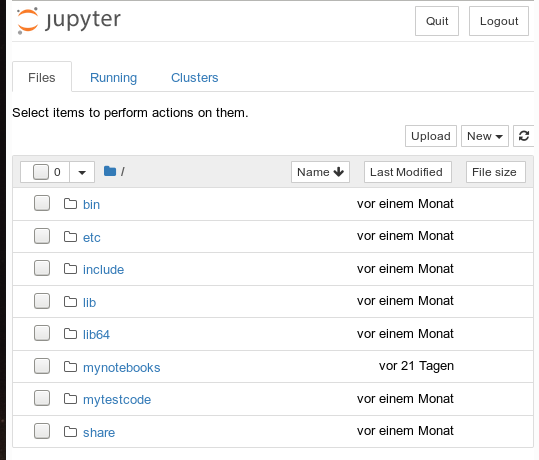Note that a standard port 8888 is used; this port should not be used by other services on your machine.

On the displayed web page we can move to the “mynotebooks” directory. We open a new notebook there by clicking on the “New”-button on the right side of the browser window:

We choose Python3 as the relevant interpreter and get a new browser window: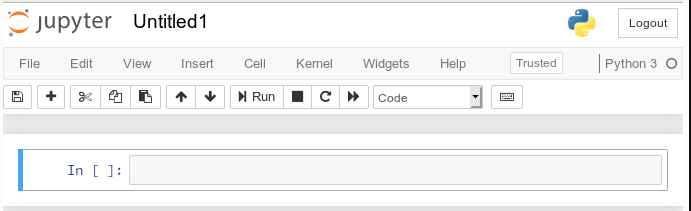We give the notebook a title by clicking on “File >> Save as …” before start using the provided input “cell” for coding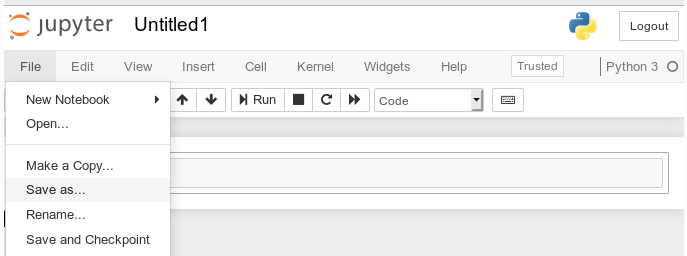I name it “moons1” in the next input form and check afterward in a terminal that the file “/projekte/GIT/ai/ml1mynotebooks/moons1.ipynb” really has been created; you see this also in the address bar of the browser – see below.

# Lets do some plotting within a notebook

Most of the icons regarding the notebook screen are self explanatory. The interesting and pretty nice thing about a Jupyter notebook is that the multiple lines of Python code can be filled into cells. All lines can be executed in a row by first choosing a cell via clicking on a it and then clicking on the “Run” button.

As a first exercise I want to do some plotting with “matplotlib” (which I also installed together with the numpy package in a previous article). We start by importing the required modules: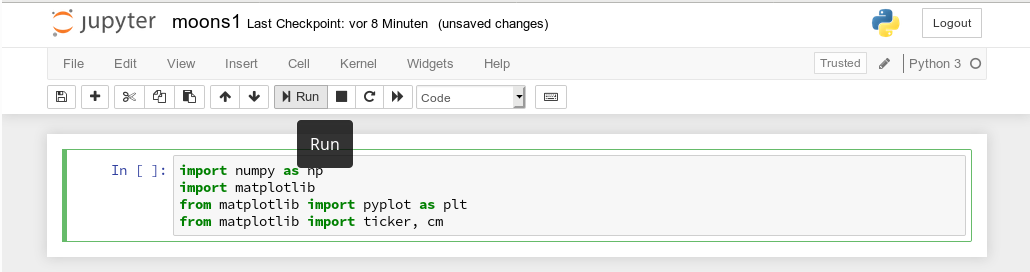A new cell for input opens automatically (it is clever to separate cells for imports and for real code). Let us produce a most simple plot there: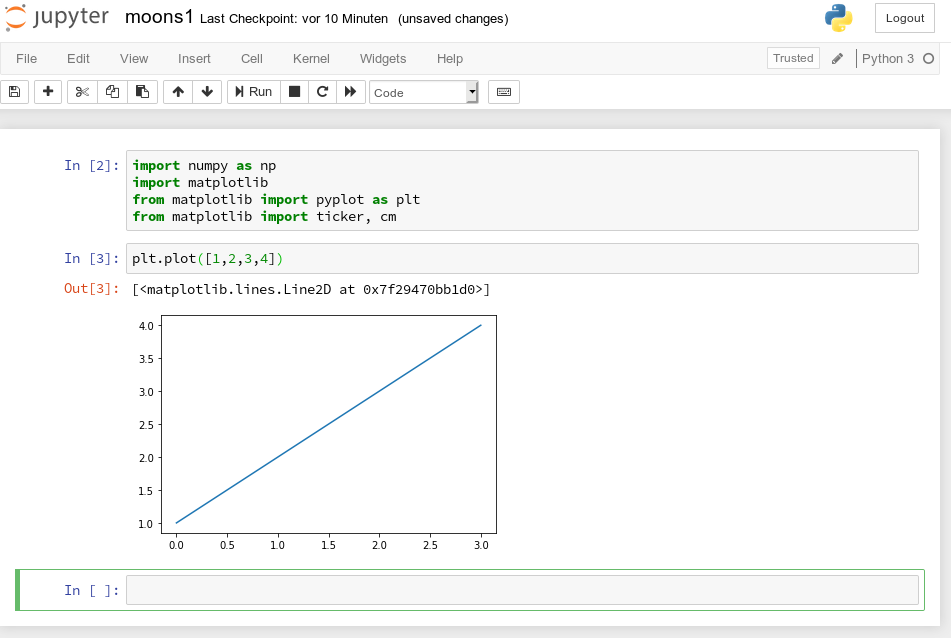No effort in comparison to what we had to do to prepare an Eclipse environment for plotting (see Eclipse, PyDev, virtualenv and graphical output of matplotlib on KDE – II). Calling plot routines simply works – no special configuration is required. Jupyter and the browser do all the work for us. We save our present 2 cells by clicking on the “Save“-icon.

# How do we plot contour lines or contour areas?

Later on we need to plot a separation line in a 2-dimensional parameter space between 2 clustered sets of data. This task is very similar to plotting a contour line. As this is a common task in math we expect matplotlib to provide some functionality for us. Our ultimate goal is to wrap this plotting functionality into a function or class which also accepts an SVM based ML-method of SciKit to prepare and evaluate the basic data first. But let us proceed step by step.

Some research on the Internet shows: The keys to contour plotting with matplotlib are the functions “contour()” and “contourf()” (matplotlib.pyplot.contourf):

contour(f)([X, Y,] Z, [levels], **kwargs)

“contour()” plots lines, only, whilst “contourf()” fills the area between the lines with some color.

Both functions accept data sets in the form of X,Y-coordinates and Z-values (e.g. defined by some function Z=f(X,Y)) at the respective points.

X and Y can be provided as 1-dim arrays; Z-values, however, must be given by a 2-dim array, such that len(X) == M is the number of columns in Z and len(Y) == N is the number of rows in Z. We cover the X,Y-plane with Z-values from bottom to top (Y, lines) and from the left to the right (X, columns).

Somewhat counter-intuitively, X and Y can also be provided as 2-dim arrays – with the same dimensionality as Z.
There is a nice function “meshgrid” (of packet numpy) which allows for the creation of e.g. a mesh of two 2-dimensional X- and separately Y-matrices. See for further information (numpy.meshgrid). Both arrays then have a (N,M)-layout (shape); as the degree of information of one coordinate is basically 1-dimensional, we do expect repeated values of either coordinate in the X-/Y-meshgrid-matrices.

The function “shape” gives us an output in the form of (N lines, M columns) for a 2-dim array. Lets apply all this and create a rectangle shaped (X,Y)-plane: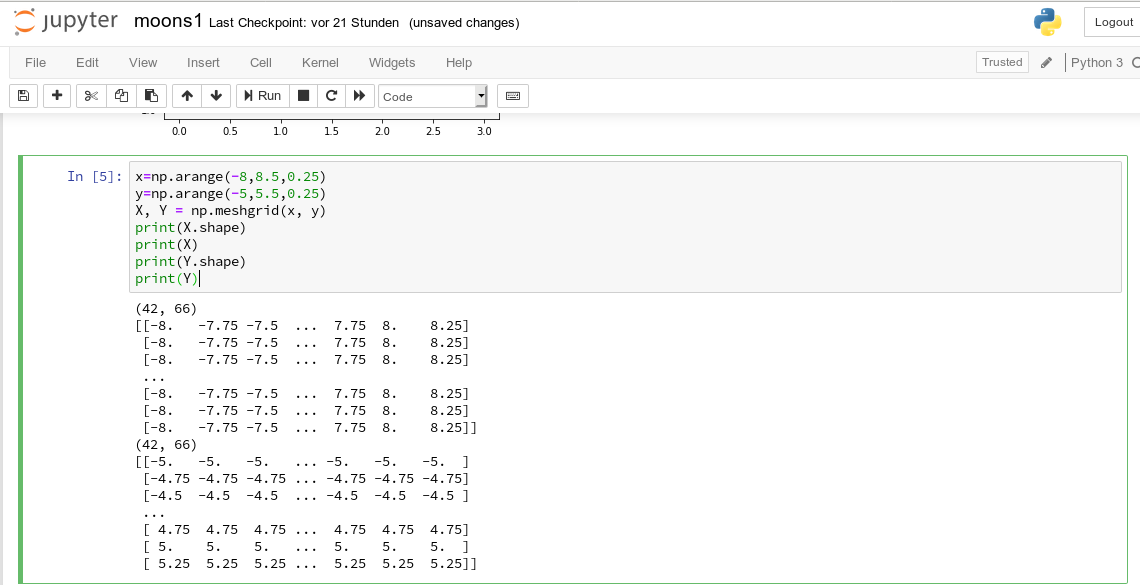The basic numpy-function “arange()” turns a range between two limiting values into an array of equally spaced values. We see that meshgrid() actually produces two 2-dim arrays of the same “shape”.

For test purposes let us use a function

Z1=-0.5* (X)**2 + 4*(Y)**2.

For this function we expect elliptical contours with the longer axis in X-direction. The “contourf()”-documentation shows that we can use the parameters “levels“, “cmap” and “alpha” to set the number of contour levels (= number of contour lines -1), a so called colormap, and the opacity of the area
coloring, respectively.

You find predefined colormaps and their names at this address: matplotlib colormaps. If you add an “_r” to the colormap-name you just reverse the color sequence.

We combine all ingredients now to create a 2D-plot (with the “plasma” colormap):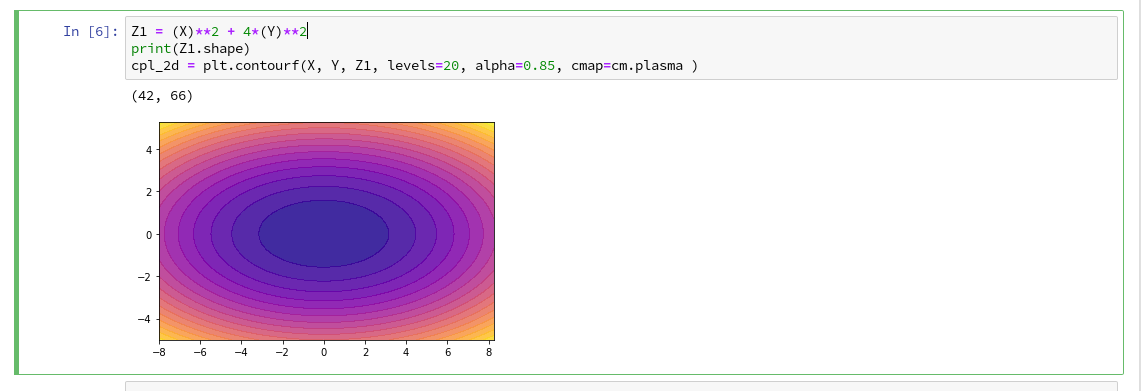Our first reasonable contour-plot within a Jupyter notebook! We got the expected elliptic curves! Time for a coffee ….

# Changing the plot size

A question that may come to your mind at this stage is: How can we change the size of the plot?

Well, this can be achieved by defining some basic parameters for plotting. You need to do this in advance of any of your specific plots. One also wants to add some labels for all axis. We, therefore, extend the code in our cell a bit by the following statements and click again on “Run”:You see that “fig_size = plt.rcParams[“figure.figsize”]” provides you with some kind of array- or object like information on the size of plots. You can change this by assigning new values to this object. “figure” is an instance of a container class for all plot elements. “plt.xlabel” and “plt.ylabel” offer a simple option to add some text to an axis of the plot.

# What about a 3D-representation …

As we are here – isn’t our function for Z1 not a good example to get a 3D-representation of our data? As 3D-plots are helpful in other contexts of ML, lets have a quick side look at this. You find some useful information at the following addresses:
PythonDataScienceHandbook and mplot3d-tutorial

I used the given information in form of the following code: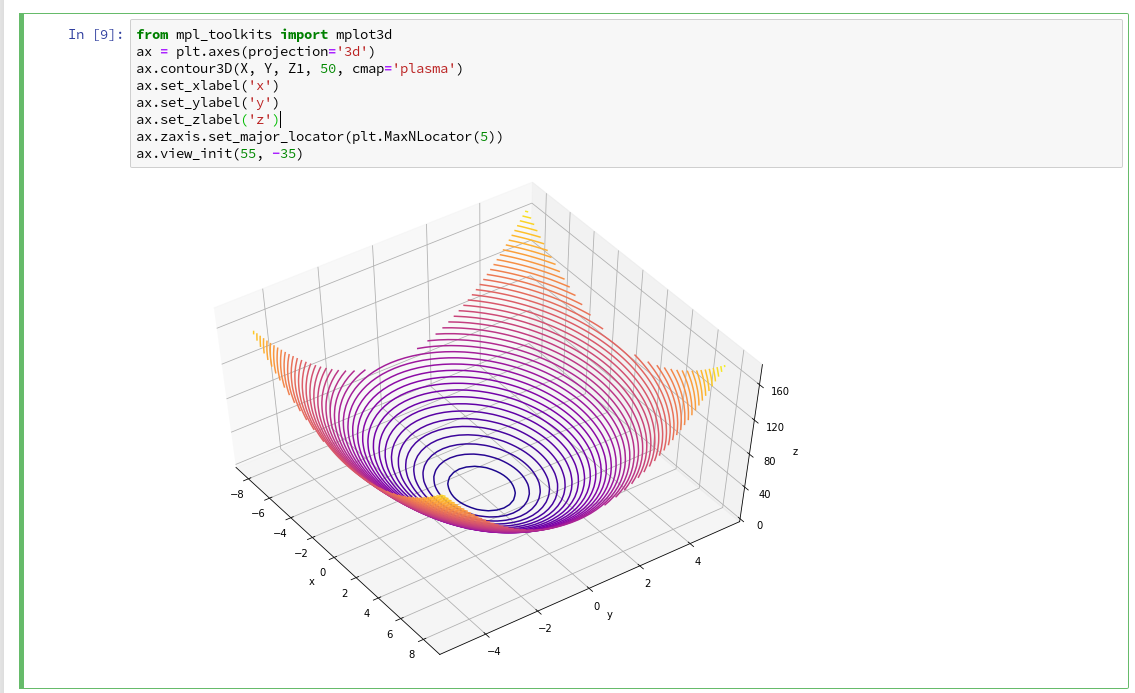You see that we can refer to a special 3D-plot-object as the output of plt.axes(projection=’3d’). The properties of such an object can be manipulated by a variety of methods. You also see that I manipulated the number of ticks on the z-axis to 5 by using a function “set_major_locator(plt.MaxNLocator(5)“. I leave it to the reader to dive deeper into manipulation options for a plot axis.

A reader asked me to show how one can set ticks and add a color-bar to the plots. I give an example code below:The result is: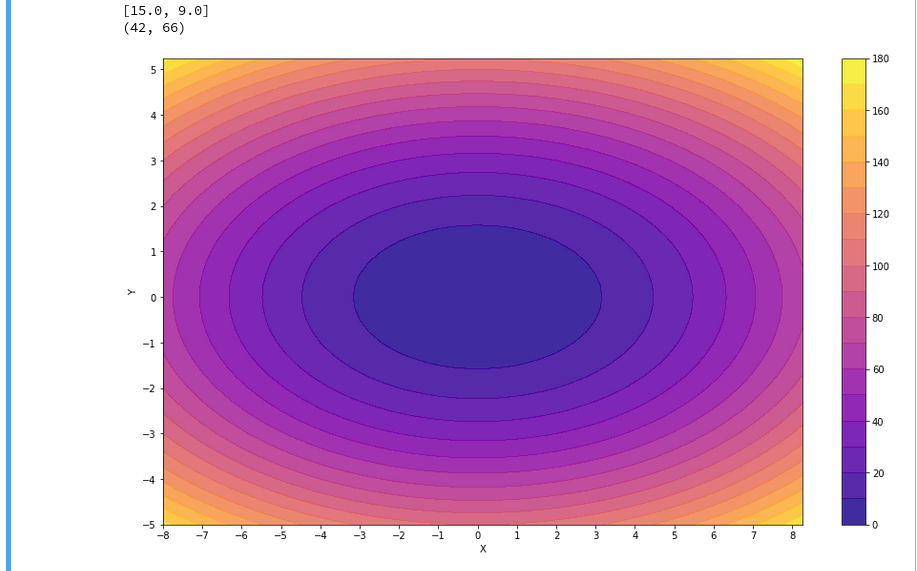For the 3D-plots we get:# Conclusion

Enough for today. We have seen that it is relatively simple to create nice contour and even 3D-plots in a Jupyter notebook environment. This new knowledge provides us with a good basis for a further approach to our objective of plotting a decision surface for the moons dataset. In the next article

The moons dataset and decision surface graphics in a Jupyter environment – III – scatter-plots and LinearSVC

we first import the moons data set into our Jupyter notebook. Then we shall create a so called “scatter plot” for all data points. Furthermore we shall train a specific SVM algorithm (LinearSVC) on the dataset.